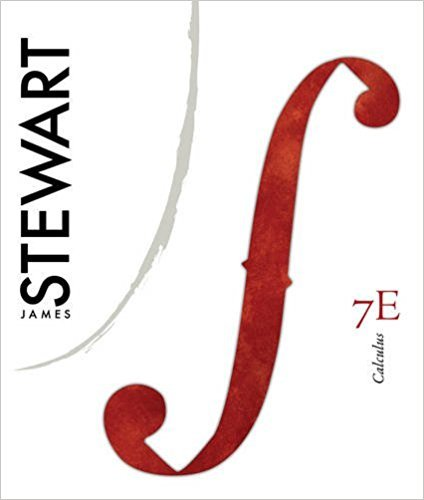×
×

# Solutions for Chapter 5: Calculus, 7th Edition## Full solutions for Calculus, | 7th Edition

ISBN: 9780538497817Solutions for Chapter 5

Solutions for Chapter 5
4 5 0 291 Reviews
26
3
##### ISBN: 9780538497817

Calculus, was written by and is associated to the ISBN: 9780538497817. This textbook survival guide was created for the textbook: Calculus,, edition: 7. Since 32 problems in chapter 5 have been answered, more than 6730 students have viewed full step-by-step solutions from this chapter. This expansive textbook survival guide covers the following chapters and their solutions. Chapter 5 includes 32 full step-by-step solutions.

Key Calculus Terms and definitions covered in this textbook
• Absolute minimum

A value ƒ(c) is an absolute minimum value of ƒ if ƒ(c) ? ƒ(x)for all x in the domain of ƒ.

• Base

See Exponential function, Logarithmic function, nth power of a.

• Coefficient of determination

The number r2 or R2 that measures how well a regression curve fits the data

• Commutative properties

a + b = b + a ab = ba

• Completing the square

A method of adding a constant to an expression in order to form a perfect square

• Difference identity

An identity involving a trigonometric function of u - v

• Distance (on a number line)

The distance between real numbers a and b, or |a - b|

• Explanatory variable

A variable that affects a response variable.

• Half-angle identity

Identity involving a trigonometric function of u/2.

• Integrable over [a, b] Lba

ƒ1x2 dx exists.

• Linear regression line

The line for which the sum of the squares of the residuals is the smallest possible

• Normal curve

The graph of ƒ(x) = e-x2/2

• Open interval

An interval that does not include its endpoints.

• Outcomes

The various possible results of an experiment.

• Paraboloid of revolution

A surface generated by rotating a parabola about its line of symmetry.

• Projectile motion

The movement of an object that is subject only to the force of gravity

• Range screen

See Viewing window.

• Slope

Ratio change in y/change in x

• Unit circle

A circle with radius 1 centered at the origin.

• z-axis

Usually the third dimension in Cartesian space.

×